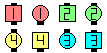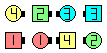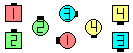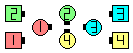Definitions of Square Dance Calls and Concepts
Ease Off [C3A]Index -->  Plus  |  A1  |  A2  |  C1  |  C2  |  C3A  |  C3B  |  C4  |  NOL  |
Definitions (Text Only) -->  Plus  |  A1  |  A2  |  C1  |  C2  |  C3A  |  C3B  |  C4  |  NOL  |
 Find call:

 \$B8@8l(B\$BJ?9T\$J(B Line \$B\$^\$?\$OB>\$NE,@Z\$J(B formation \$B\$+\$i!%(B

Outsides \$B\$O(B Zing \$B\$r(B, Centers \$B\$O(B (Concentric) Circulate \$B\$r\$7(B Face In \$B\$r\$7\$^\$9!%(B

\$BJ?9T\$J(B Line \$B\$+\$i\$O(B Double Pass Thru \$B\$G=*\$o\$j\$^\$9!%(BEase Off\$B\$NA0(B \$B8e(BEase Off\$B\$NA0(B \$B8e(B

Zing [C1] (Jim Davis 1981): \$BE,@Z\$J(B 2 x 2 \$B\$+\$i!%(BLeaders \$B\$O(B, 1 \$B\$D\$NF0\$-\$H\$7\$F(B, 1/4 Out - Run \$B\$r(B, Trailers \$B\$O(B Box Circulate - 1/4 In \$B\$r\$7\$^\$9!%(BT-Bone \$B\$G\$J\$\$(B 2 x 2 \$B\$+\$i\$O(B, \$B8~\$+\$\$9g\$C\$?%+%C%W%k\$G=*\$o\$j\$^\$9!%(B

Nice & Easy [C4]: \$BE,@Z\$J(B formation \$B\$+\$i!%(BOutsides \$B\$O(B Zing \$B\$r(B, Centers \$B\$O(B Trade & Roll \$B\$r\$7\$^\$9!%(B

Wahoo [obsolete]: 2 x 2 \$B\$+\$i!%(BBox Circulate & 1/4 In \$B\$r\$7\$^\$9!%\$3\$l\$O(B, Ease Off \$B\$N(B centers \$B\$N%Q!<%H\$G\$9!%(BCALLERLAB definition for Ease OffChoreography for Ease OffComments? Questions? Suggestions?